Next: Minimal and maximal real Up: Simplification procedures Previous: The KharitonovConsistency procedure   Contents

### The WeylFilter procedure

Let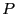be a polynomial and be maxroot the maximal modulus of the root of. Fromwe may derive a the unitary polynomial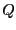such that the roots ofhave a modulus lower or equal to 1 and if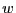is a root ofthen maxrootis a root of.

Let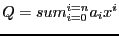which may also be written as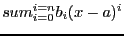where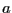is some fixed point.

Let a range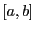for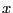and let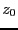be the mid point of the range. We consider the square in the complex plane centered atand whose edge length is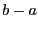. Let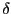be the length of the half-diagonal of this square. If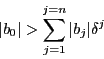then the polynomial has no root in the square. For a given list of equations we consider in turn each equation and determine if it may be considered as a parametric polynomial. For example the equation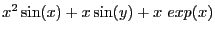will be considered as a second order polynomial inwith coefficients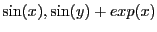(but not a polynomial in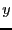). The procedure

WeylFilter(Func,Vars,FullVars,MaxRoot,TypeB,name)

will consider each equation in the list Func and examine if it may considered as a parametric polynomial successively in each variable in the list Vars. If yes the Weyl filter will be used on the polynomial whose coefficients are functions of the variables in the list FullVars (all variables in Vars must be a member of FullVars). MaxRoot is a list which indicates for each variable in Vars what is the maximum modulus of the roots of all parametric polynomials in this variable. An element of MaxRoot may be a numerical value of the key-word "automatic" which indicates that the C++ program will try to determine the maximal modulus. The list TypeB indicates for each variable in Vars how are computed the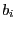i.e. numerically with the keyword "numeric", or symbolically (which is usually more efficient) with the keyword "symbolic". The simplification procedure will be named name and be written in the file name.C. For the previous example the procedure will be

WeylFilter([x^2*sin(x)+x*sin(y)+ x*exp(x)],[x],[x,y],["automatic"],["symbolic"],"SIMP");

Another example of the use of this procedure is presented in section 12.3.Next: Minimal and maximal real Up: Simplification procedures Previous: The KharitonovConsistency procedure   Contents
Jean-Pierre Merlet 2012-12-20